# Resources tagged with: GeoGebra

Filter by: Content type:
Age range:
Challenge level:

### There are 90 results

Broad Topics > Physical and Digital Manipulatives > GeoGebra### Tessellation Interactivity

##### Age 7 to 16Challenge Level

An environment that enables you to investigate tessellations of regular polygons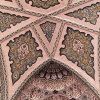### Angles Inside

##### Age 11 to 14Challenge Level

Draw some angles inside a rectangle. What do you notice? Can you prove it?##### Age 14 to 16Challenge Level

Make five different quadrilaterals on a nine-point pegboard, without using the centre peg. Work out the angles in each quadrilateral you make. Now, what other relationships you can see?### Bow Tie

##### Age 11 to 14Challenge Level

Show how this pentagonal tile can be used to tile the plane and describe the transformations which map this pentagon to its images in the tiling.### Semi-regular Tessellations

##### Age 11 to 16Challenge Level

Semi-regular tessellations combine two or more different regular polygons to fill the plane. Can you find all the semi-regular tessellations?### Polygon Rings

##### Age 11 to 14Challenge Level

Join pentagons together edge to edge. Will they form a ring?### Using Geogebra

##### Age 11 to 18### Right Angles

##### Age 11 to 14Challenge Level

Can you make a right-angled triangle on this peg-board by joining up three points round the edge?### Subtended Angles

##### Age 11 to 14Challenge Level

What is the relationship between the angle at the centre and the angles at the circumference, for angles which stand on the same arc? Can you prove it?### The Medieval Octagon

##### Age 14 to 16Challenge Level

Medieval stonemasons used a method to construct octagons using ruler and compasses... Is the octagon regular? Proof please.### Same Length

##### Age 11 to 16Challenge Level

Construct two equilateral triangles on a straight line. There are two lengths that look the same - can you prove it?### Triangles in Circles

##### Age 11 to 14Challenge Level

Can you find triangles on a 9-point circle? Can you work out their angles?### Points in Pairs

##### Age 14 to 16Challenge Level

Move the point P to see how P' moves. Then use your insights to calculate a missing length.##### Age 11 to 16Challenge Level

Draw some quadrilaterals on a 9-point circle and work out the angles. Is there a theorem?### Squaring the Circle and Circling the Square

##### Age 14 to 16Challenge Level

If you continue the pattern, can you predict what each of the following areas will be? Try to explain your prediction.### Strolling Along

##### Age 14 to 18Challenge Level

What happens when we multiply a complex number by a real or an imaginary number?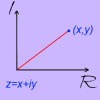### A Brief Introduction to the Argand Diagram

##### Age 14 to 18Challenge Level

Complex numbers can be represented graphically using an Argand diagram. This problem explains more...### Opening the Door

##### Age 14 to 18Challenge Level

What happens when we add together two complex numbers?### Into the Wilderness

##### Age 14 to 18Challenge Level

Let's go further and see what happens when we multiply two complex numbers together!### Mapping the Territory

##### Age 14 to 18Challenge Level

Can you devise a system for making sense of complex multiplication?### Where Is the Dot?

##### Age 14 to 16Challenge Level

A dot starts at the point (1,0) and turns anticlockwise. Can you estimate the height of the dot after it has turned through 45 degrees? Can you calculate its height?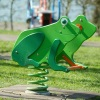### Surprising Equalities

##### Age 14 to 18Challenge Level

Take any triangle, and construct squares on each of its sides. What do you notice about the areas of the new triangles formed?### Polar Coordinates

##### Age 14 to 18

Cartesian Coordinates are not the only way!### Rolling Around

##### Age 11 to 14Challenge Level

A circle rolls around the outside edge of a square so that its circumference always touches the edge of the square. Can you describe the locus of the centre of the circle?### Mixing More Paints

##### Age 14 to 16Challenge Level

Can you find an efficent way to mix paints in any ratio?### L-triominoes

##### Age 14 to 16Challenge Level

L triominoes can fit together to make larger versions of themselves. Is every size possible to make in this way?### Solving Together - Estimating Angles

##### Age 11 to 14

Week 2
How well can you estimate angles? Playing this game could improve your skills.### Mixing Paints

##### Age 11 to 14Challenge Level

Can you work out how to produce different shades of pink paint?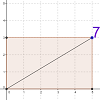### Exploring Diagonals

##### Age 11 to 16

Move the corner of the rectangle. Can you work out what the purple number represents?### Tilting Triangles

##### Age 14 to 16Challenge Level

A right-angled isosceles triangle is rotated about the centre point of a square. What can you say about the area of the part of the square covered by the triangle as it rotates?### Sine and Cosine for Connected Angles

##### Age 14 to 16Challenge Level

The length AM can be calculated using trigonometry in two different ways. Create this pair of equivalent calculations for different peg boards, notice a general result, and account for it.### Impossible Picture?

##### Age 14 to 16Challenge Level

Under what circumstances can you rearrange a big square to make three smaller squares?### Squirty

##### Age 14 to 16Challenge Level

Using a ruler, pencil and compasses only, it is possible to construct a square inside any triangle so that all four vertices touch the sides of the triangle.##### Age 14 to 16Challenge Level### Sine and Cosine

##### Age 14 to 16Challenge Level

The sine of an angle is equal to the cosine of its complement. Can you explain why and does this rule extend beyond angles of 90 degrees?##### Age 14 to 16Challenge Level

The points P, Q, R and S are the midpoints of the edges of a non-convex quadrilateral.What do you notice about the quadrilateral PQRS and its area?### Estimating Angles

##### Age 7 to 14Challenge Level

How good are you at estimating angles?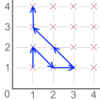### Coordinates of Corners

##### Age 11 to 16

Use the applet to make some squares. What patterns do you notice in the coordinates?### Areas from Vectors

##### Age 11 to 16

Use the applet to explore the area of a parallelogram and how it relates to vectors.### Pythagoras Proofs

##### Age 14 to 16Challenge Level

Can you make sense of these three proofs of Pythagoras' Theorem?### Dice/spinner Interactives

##### Age 11 to 14Challenge Level### Round and Round a Circle

##### Age 14 to 16Challenge Level

Can you explain what is happening and account for the values being displayed?### Shear Magic

##### Age 11 to 14Challenge Level

Explore the area of families of parallelograms and triangles. Can you find rules to work out the areas?### Reflecting Squarely

##### Age 11 to 14Challenge Level

In how many ways can you fit all three pieces together to make shapes with line symmetry?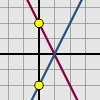### Reflecting Lines

##### Age 11 to 14Challenge Level

Investigate what happens to the equations of different lines when you reflect them in one of the axes. Try to predict what will happen. Explain your findings.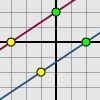### Translating Lines

##### Age 11 to 14Challenge Level

Investigate what happens to the equation of different lines when you translate them. Try to predict what will happen. Explain your findings.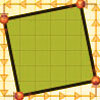### At Right Angles

##### Age 14 to 16Challenge Level

Can you decide whether two lines are perpendicular or not? Can you do this without drawing them?### Triangle in a Triangle

##### Age 14 to 16Challenge Level

Can you work out the fraction of the original triangle that is covered by the inner triangle?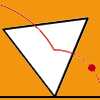### Speeding Up, Slowing Down

##### Age 11 to 14Challenge Level

Experiment with the interactivity of "rolling" regular polygons, and explore how the different positions of the red dot affects its speed at each stage.### Rollin' Rollin' Rollin'

##### Age 11 to 14Challenge Level

Two circles of equal radius touch at P. One circle is fixed whilst the other moves, rolling without slipping, all the way round. How many times does the moving coin revolve before returning to P?